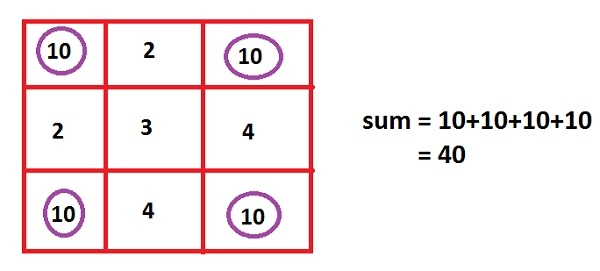Print the corner elements and their sum in a 2-D matrix in C Program.

CServer Side ProgrammingProgramming

Given an array of size 2X2 and the challenge is to print the sum of all the corner elements stored in an array.

Assume a matrix mat[r][c], with some row “r” and column “c” starting row and column from 0, then its corner elements will be; mat, mat[c-1], mat[r-1], mat[r-1][c-1]. Now the task is to get these corner elements and sum those corner elements i.e., mat + mat[c-1] + mat[r-1] + mat[r-1][c-1], and print the result on the screen.

Example

Input: Enter the matrix elements :
10 2 10
2 3 4
10 4 10
Output: sum of matrix is : 40Algorithm

START
Step 1-> create macro for rows and column as #define row 3 and #define col 3
Step 2 -> main()
Declare int sum=0 and array as a[row][col] and variables int i,j,n
Loop For i=0 and i<3 and i++
Loop For j=0 and j<3 and j++
Input a[i][j]
End
End
Print  + a[row-1] +a[col-1] + a[col-1][row-1]
STOP

Example

#include<stdio.h>
#define row 3
#define col 3
int main(){
int sum=0,a[row][col],i,j,n;
printf("Enter the matrix elements : ");
for(i=0;i<3;i++){
for(j=0;j<3;j++){
scanf("%d",&a[i][j]);
}
}
printf("sum of matrix is : %d",a + a[row-1] +a[col-1] + a[col-1][row-1] );
return 0;
}

Output

if we run above program then it will generate following output

Enter the matrix elements :
10 2 10
2 3 4
10 4 10
sum of matrix is : 40